## The vertex of this parabola is at (-2,-3). When the y-value is -2, the x-value is -5. What is the coefficient of the squared term in the par

Question

The vertex of this parabola is at (-2,-3). When the y-value is -2, the x-value is -5. What is the coefficient of the squared term in the parabola’s equation? 5 re (-2, -3)​

in progress 0
1 month 2021-08-12T07:50:31+00:00 1 Answers 4 views 0

The coefficient of the squared term of the equation is 1/9.

Step-by-step explanation:

We are given that the vertex of the parabola is at (-2, -3). We also know that when the y-value is -2, the x-value is -5. Using this information we want to find the cofficient of the squared term in the parabola’s equation.

Since we are given the vertex, we can use the vertex form: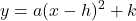Where a is the leading coefficient and (h, k) is the vertex.

Since the vertex is (-2, -3), h = -2 and k = -3: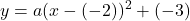Simplify: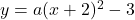We are also given that y = -2 when x = -5. Substitute: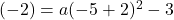Solve for a. Simplify: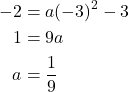Therefore, our full vertex equation is: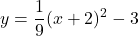We can expand: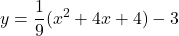Simplify: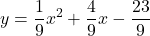The coefficient of the squared term of the equation is 1/9.